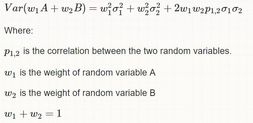Choose Language Hide Translation Bar
Highlighted

## How to calculate the variance of the weighted sum of >10 components

Perl https://stats.stackexchange.com/questions/5392/variance-of-two-weighted-random-variables; the variance of A+B is:Thus, one would imagine that the calculation of the weighted sum of >10 components will be very very complicated. Was wondering whether JMP has an easy way to perform the calculation. Thanks!

5 REPLIES 5
Highlighted

## Re: How to calculate the variance of the weighted sum of >10 components

The formula quoted in your reference is the one used to estimate the variance of a calculated variable. In the classic Box, Hunter and Hunter text "Statistics for Experimenters"  and older texts it was referred to as transmission of errors. Note, for the general case, the weights are not required to sum to 1.

The formula can get long for many variables but it can be simplified using matrix algebra.

If you have actual data, it is easy to calculate in JMP.  See the script below.

``````Names Default to Here(1);

dt = Open("\$Sample_Data/Iris.jmp"); //a data file with 4 variables and mixed correlation

x = dt << get as matrix;

w = [0.2 0.2 0.4 0.2];

cov =  Covariance(x, <<"Pairwise");
wcov = Emult(w`*w, cov); //element wise multiplication

show(cov,wcov,sum(wcov));       /* log: Sum(wcov) = 0.943490362416108;  */

//note the diagonal of wcov will be added once and each off diagonal twice, sum(wcov) will be the weighted variance

//now compute the weighted sum and find its variance, that is the std dev squared

dt << New Column("WSum", numeric, Continuous, <<set Each Value(0.2 * :Sepal length + 0.2 * :Sepal width + 0.4 * :Petal length + 0.2 * :Petal width ));

show( (Col Std Dev(:WSum))^2 ); /* log: Col Std Dev(:WSum) ^ 2 = 0.943490362416108; */``````
Highlighted

## Re: How to calculate the variance of the weighted sum of >10 components

Thanks much for the reply and solution! Given that the provided example doesn't quite match the situation I am dealing with, I have are two additional questions with simplified data set in the attachment.

As shown by the attached xls, there are 5 different components (test cases) with different weights. Each test case has different number of measurements for its corresponding power consumption (in mA) .

Questions:

1. How would you calculate the power consumption of the weighted sum?

My approach is to use the mean (or median) power consumption of multiple measurements of each test case and then sum them up according to the weights.

2. How would you calculate the variance of the weighted sum?

Highlighted

## Re: How to calculate the variance of the weighted sum of >10 components

Forgot to provide the covariance matrix for these 5 test cases. Updated xls with both correlation and covariance matrix.

BTW, the correlation and covariance matrix were generated based upon the mean power consumption of each test case over multiple measurements on 20 different devices.

Highlighted

## Re: How to calculate the variance of the weighted sum of >10 components

How was the covariance matrix reported in Excel computed?  It should be  (X-mu)`*X-mu)/ (n-1).

This covariance in Excel looks strange to me.

I still do not understand your data:

• The 5 values for CR and BD are identical??
• What does measurement ID represent? Why do some have 5 and others 23? Does this represent multiple measurements within a component on a single device?
• Can you provide the 20 x 5 table of your calculated results used to calculate the Covariance matrix?

Note:  The 20 device results used to compute the covariance should represent random variation. From past experience, I have seen attempts to use 20 components that represent spatial variation and not representative of the target population of devices. Since this is getting out of the realm of JMP capability, a private message might be more appropriate to continue discussion.

Below is an example of matrix manipulation to compute pairwise covariance using JMP matrix functions.

``````Names Default to Here(1);

dt = Open("\$Sample_Data/Iris.jmp"); //a data file with 4 variables and mixed correlation

x = dt << get as matrix;

xb = emult( J(nrow(dt), ncol(x), 1) , V Mean(x) );
xc = x-xb;
cov =  Covariance(x);
show( (xc`*xc)/(nrow(dt)-1) , cov);
/*: Log Output

(xc` * xc) / (N Row(dt) - 1) =
[	0.685693512304251 -0.0424340044742729 1.27431543624161 0.516270693512304,
-0.0424340044742729 0.189979418344519 -0.329656375838926 -0.12163937360179,
1.27431543624161 -0.329656375838926 3.11627785234899 1.29560939597315,
0.516270693512304 -0.12163937360179 1.29560939597315 0.581006263982103];
cov =
[	0.685693512304251 -0.0424340044742729 1.27431543624161 0.516270693512304,
-0.0424340044742729 0.189979418344519 -0.329656375838926 -0.12163937360179,
1.27431543624161 -0.329656375838926 3.11627785234899 1.29560939597315,
0.516270693512304 -0.12163937360179 1.29560939597315 0.581006263982103];
*/``````

Highlighted

## Re: How to calculate the variance of the weighted sum of >10 components

Thanks and agreed that a private message might be more appropriate to continue discussion! Will send you a private message shortly.

Article Labels

There are no labels assigned to this post.